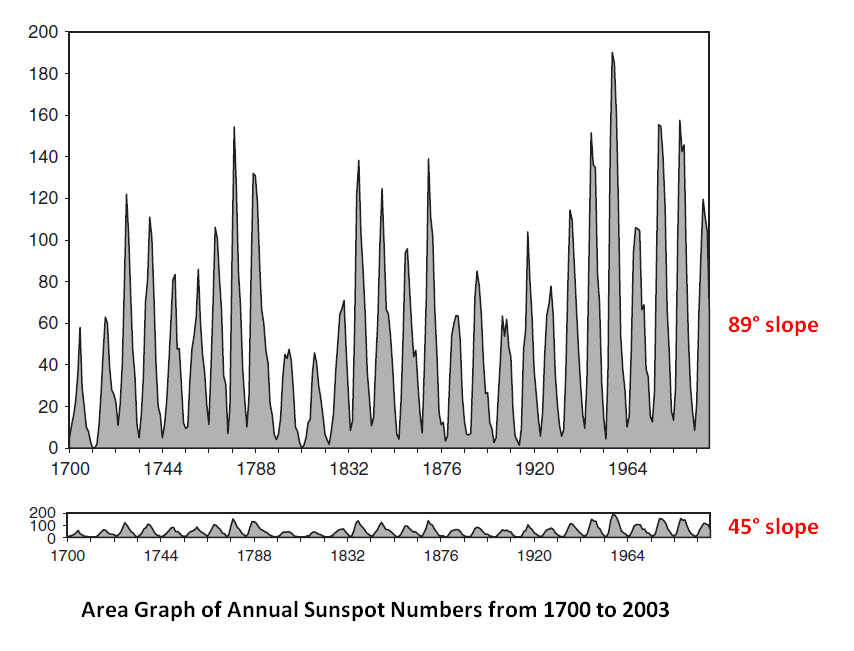# Rate of Change

In applications,

• the functions usually represent physical quantities,
• the derivatives represent their rates of change,
• and the equation defines a relationship between the two.
• The derivative measures the instantaneous rate of change of the function, as distinct from its average rate of change.
• The aspect ratio of a graph is an important factor for judging rate of change.

## Documentation / Reference

Discover MoreData Viz - (Timing|Easing) Function

Timing function (also known as easing) are used in transition to calculate the speed and smooth of the animation between state. They specify the rate of change of a parameter over time. Objects in real...Math - Derivative (Sensitivity to Change, Differentiation)

The derivative of a function of a real variable measures the sensitivity to change of a quantity (a function or dependent variable) which is determined by another quantity (the independent variable). ...Alerting is the science of detection Deviations from prediction on Time serie are a powerful way to tell when there is a problem and to trigger alerts when a is reached. See also: ...Viz - (Change|Variation)

Change of value for one variable. Changes are a series of visual comparison. See also: RateViz - Graph Aspect Ratio

The aspect ratio of a graph between the height and width of the axis. rate of change0963488414The Elements of Graphing Data (1994) Viewers can best understand changes in the slope of the line when...